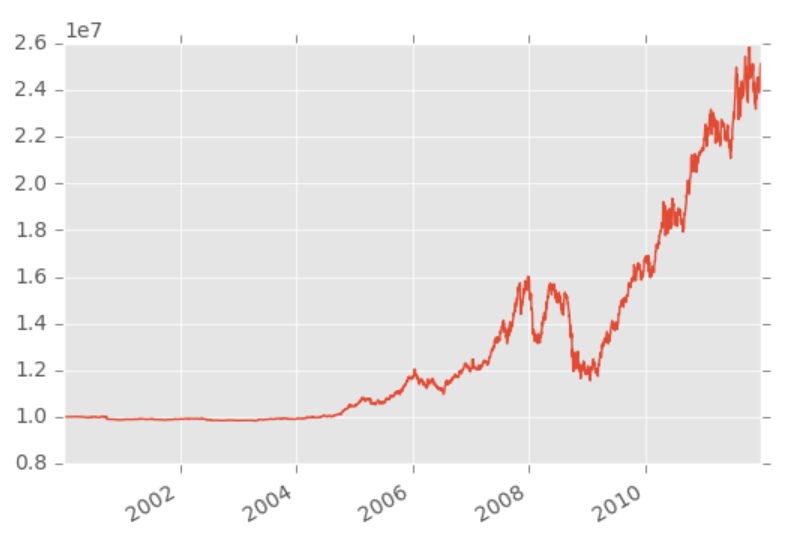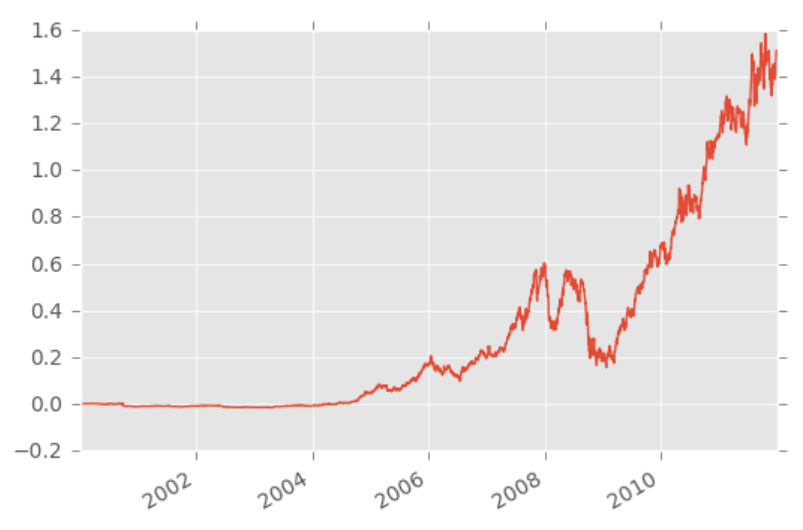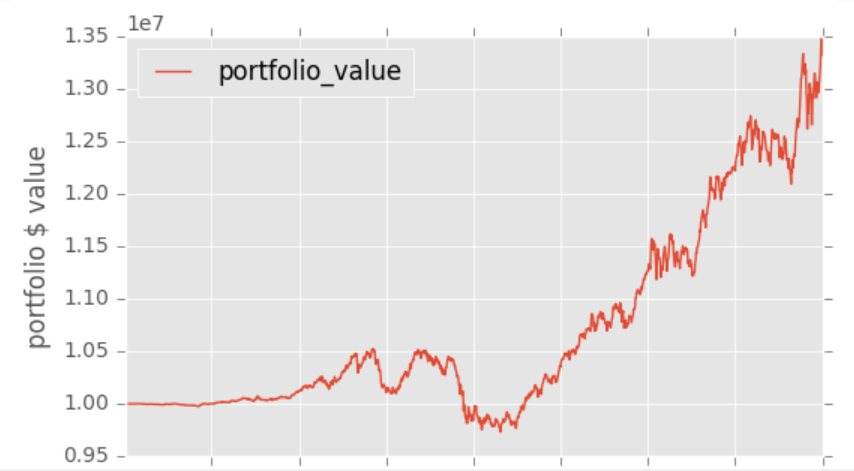## Zipline backtest visualization - Python Programming for Finance p.26

Welcome to part 2 of the local backtesting with Zipline tutorial series. In the previous tutorial, we've installed Zipline and run a backtest, seeing that the return is a dataframe with all sorts of information for us. We're going to now see how we can interact with this to visualize our results.

Up to this point, our script has the following:

Required IPython magic for zipline:

`%load_ext zipline`

Then:

```from zipline.api import order, record, symbol

def initialize(context):
pass

def handle_data(context, data):
order(symbol('AAPL'), 10)
record(AAPL=data.current(symbol('AAPL'), 'price'))```

To run, we used:

`%zipline --bundle quantopian-quandl --start 2000-1-1 --end 2012-1-1 -o backtest.pickle`, you also could use `zipline.exe` to run things. The return here is a pandas dataframe, which we also stored to `backtest.pickle`.

Now, we have a few options. One is to just load in the dataframe and visualize it. For example:

```import pandas as pd
import matplotlib.pyplot as plt
from matplotlib import style

style.use('ggplot')

backtest_df.portfolio_value.plot()
plt.show()```That's just one quick example. We can also do:

`backtest_df.columns`

...seeing that we have quite a few things that are automatically tracked for us:

```Index(['AAPL', 'algo_volatility', 'algorithm_period_return', 'alpha',
'benchmark_period_return', 'benchmark_volatility', 'beta',
'capital_used', 'ending_cash', 'ending_exposure', 'ending_value',
'excess_return', 'gross_leverage', 'long_exposure', 'long_value',
'longs_count', 'max_drawdown', 'max_leverage', 'net_leverage', 'orders',
'period_close', 'period_label', 'period_open', 'pnl', 'portfolio_value',
'positions', 'returns', 'sharpe', 'short_exposure', 'short_value',
'shorts_count', 'sortino', 'starting_cash', 'starting_exposure',
'treasury_period_return'],
dtype='object')```

Just like in Quantopian, we can also use the `record` function to record more data to our output. We can also perform modifications to the dataframe, something like:

```backtest_df.portfolio_value.pct_change().fillna(0).add(1).cumprod().sub(1).plot()
plt.show()```Another option you have to visualize things is automatically with an `analyze` function.

```from zipline.api import order, record, symbol
import matplotlib.pyplot as plt
from matplotlib import style

style.use('ggplot')

def initialize(context):
pass

def handle_data(context, data):
order(symbol("AAPL"), 10)
record(AAPL=data.current(symbol('EBAY'), 'price'))

def analyze(context, perf):
fig = plt.figure()
perf.portfolio_value.plot(ax=ax1)
ax1.set_ylabel('portfolio \$ value')
plt.legend(loc=0)
plt.show()    ```
`%zipline --bundle quantopian-quandl --start 2001-1-1 --end 2005-1-1 -o backtest.pickle`I have personally found that I prefer to keep the backtest and visualization separated, so I can tinker around with the results, rather than needing to re-run everything just to visualize various different things, but, once you have solidified exactly what you want to see, the `analyze` function could save some time.

Anyway, up to this point, we've just used some pre-bundled data, and one of the major reasons why we might want to use Zipline locally is to use our own data, so let's work on doing that in the next tutorial!

The next tutorial:• Intro and Getting Stock Price Data - Python Programming for Finance p.1

• Handling Data and Graphing - Python Programming for Finance p.2

• Basic stock data Manipulation - Python Programming for Finance p.3

• More stock manipulations - Python Programming for Finance p.4

• Automating getting the S&P 500 list - Python Programming for Finance p.5

• Getting all company pricing data in the S&P 500 - Python Programming for Finance p.6

• Combining all S&P 500 company prices into one DataFrame - Python Programming for Finance p.7

• Creating massive S&P 500 company correlation table for Relationships - Python Programming for Finance p.8

• Preprocessing data to prepare for Machine Learning with stock data - Python Programming for Finance p.9

• Creating targets for machine learning labels - Python Programming for Finance p.10 and 11

• Machine learning against S&P 500 company prices - Python Programming for Finance p.12

• Testing trading strategies with Quantopian Introduction - Python Programming for Finance p.13

• Placing a trade order with Quantopian - Python Programming for Finance p.14

• Scheduling a function on Quantopian - Python Programming for Finance p.15

• Quantopian Research Introduction - Python Programming for Finance p.16

• Quantopian Pipeline - Python Programming for Finance p.17

• Alphalens on Quantopian - Python Programming for Finance p.18

• Back testing our Alpha Factor on Quantopian - Python Programming for Finance p.19

• Analyzing Quantopian strategy back test results with Pyfolio - Python Programming for Finance p.20

• Strategizing - Python Programming for Finance p.21

• Finding more Alpha Factors - Python Programming for Finance p.22

• Combining Alpha Factors - Python Programming for Finance p.23

• Portfolio Optimization - Python Programming for Finance p.24

• Zipline Local Installation for backtesting - Python Programming for Finance p.25

• Zipline backtest visualization - Python Programming for Finance p.26
• Custom Data with Zipline Local - Python Programming for Finance p.27

• Custom Markets Trading Calendar with Zipline (Bitcoin/cryptocurrency example) - Python Programming for Finance p.28